# NCERT Solutions for Class 10 Maths Chapter 6 Triangles Ex 6.6

Get Free NCERT Solutions for Class 10 Maths Chapter 6 Ex 6.6 PDF. Triangles Class 10 Maths NCERT Solutions are extremely helpful while doing your homework. Exercise 6.6 Class 10 Maths NCERT Solutions were prepared by Experienced ncert-books.in Teachers. Detailed answers of all the questions in Chapter 6 Maths Class 10 Triangles Exercise 6.6 provided in NCERT TextBook.

## NCERT Solutions for Class 10 Maths Chapter 6 Triangles Ex 6.6

NCERT Solutions for Class 10 Maths Chapter 6 Triangles Ex Ex 6.6 are part of NCERT Solutions for Class 10 Maths. Here we have given NCERT Solutions for Class 10 Maths Chapter 6 Triangles Exercise 6.6

Question 1.
In the given figure, PS is the bisector of ∠QPR of ∆PQR. Prove that$\frac { QS }{ SR } =\frac { PQ }{ PR }$Solution: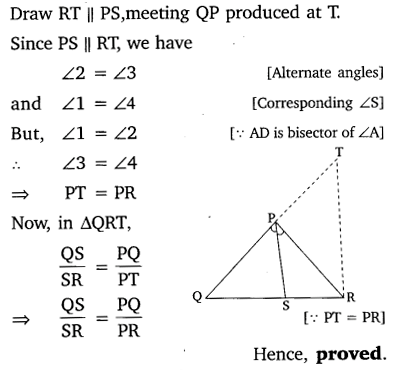Question 2.
In the given figure, D is a point on hypotenuse AC of ∆ABC, DM ⊥ BC and DN ⊥ AB. Prove that:
(i) DM2 = DN X MC
(ii) DN2 = DM X ANSolution: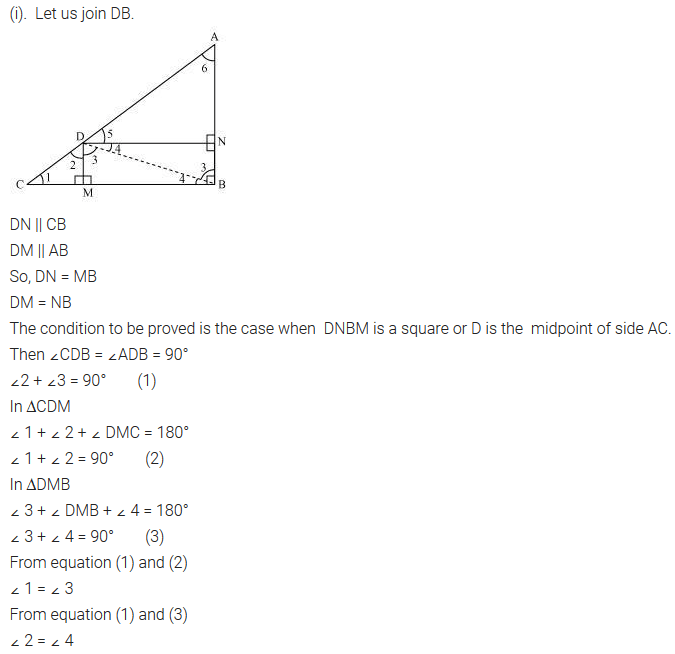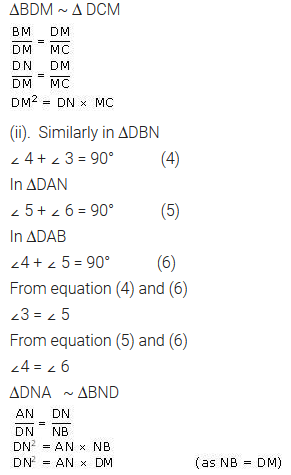Question 3.
In the given figure, ABc is triangle in which ∠ABC > 90° and AD ⊥ CB produced. Prove that AC2 = AB2 + BC2  + 2BC X BDSolution: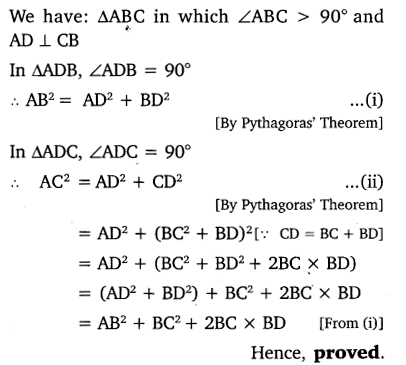Question 4.
In the given figure, ABC is atriangle in which ∠ABC 90° and AD ⊥ CB. Prove that AC2 = AB2 + BC2 – 2BC X BDSolution: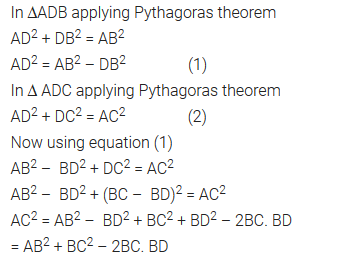Question 5.
In the given figure, Ad is a median of a triangle ABC and AM ⊥ BC. Prove thatSolution: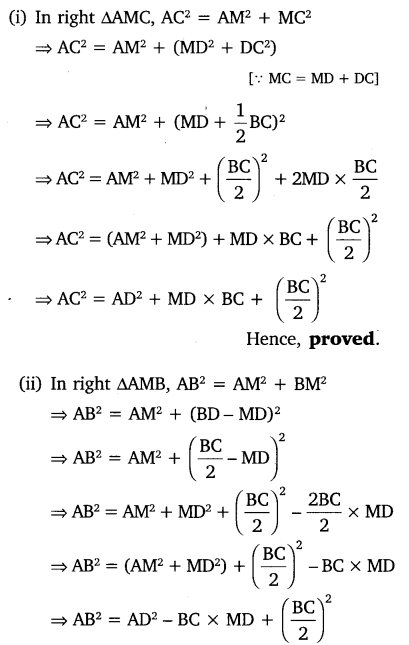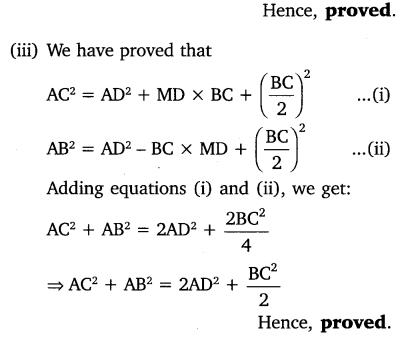Question 6.
Prove that the sum of the squares of the diagonals of parallelogram is equal to the sum of the squares of its sides.
Solution: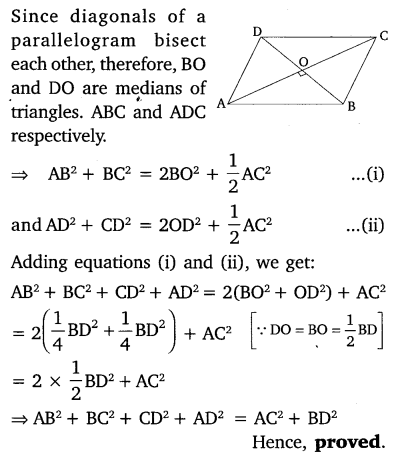Question 7.
In the given figure, two chords AB and CD intersect each other at the point P. Prove that:
(i) ∆APC ~∆DPB
(ii) AP X PB = CP X DPSolution: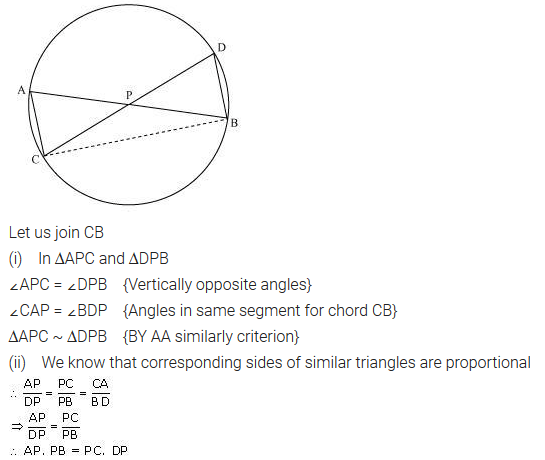Question 8.
In the given figure, two chords Ab and CD of a circle intersect each other at the point P (when produced) outside the circle. Prove that:
(i) ∆PAC ~ ∆PDB
(ii)PA X PB = PC X PDSolution:Question 9.
In the given figure, D is a point on side BC of ∆ABC, such that$\frac { BD }{ CD } =\frac { AB }{ A{ C }^{ \bullet } }$Prove that AD is the bisector of ∆BAC.Solution:Question 10.
Nazima is fly fishing in a stream. The trip of her fishing rod is 1.8m above the surface of the water and the fly at the end of the string rests on the water 3.6m away and 2.4 m from a point directly under the trip of the rod. Assuming that her string (from the trip of the rod to the fly) is that, how much string does she have out (see the figure)? If she pills in the string at the rate of 5 cm per second, what will be the  horizontal distance of the fly from her after 12 seconds?Solution:error: Content is protected !!
+